# Jim's GameDev Blog# 各向异性的头发渲染

2016-8-6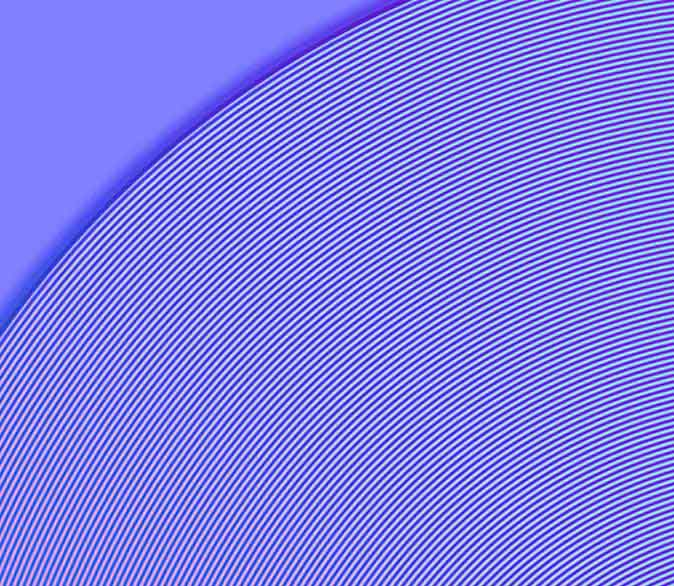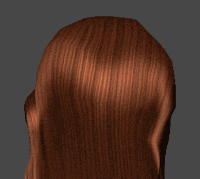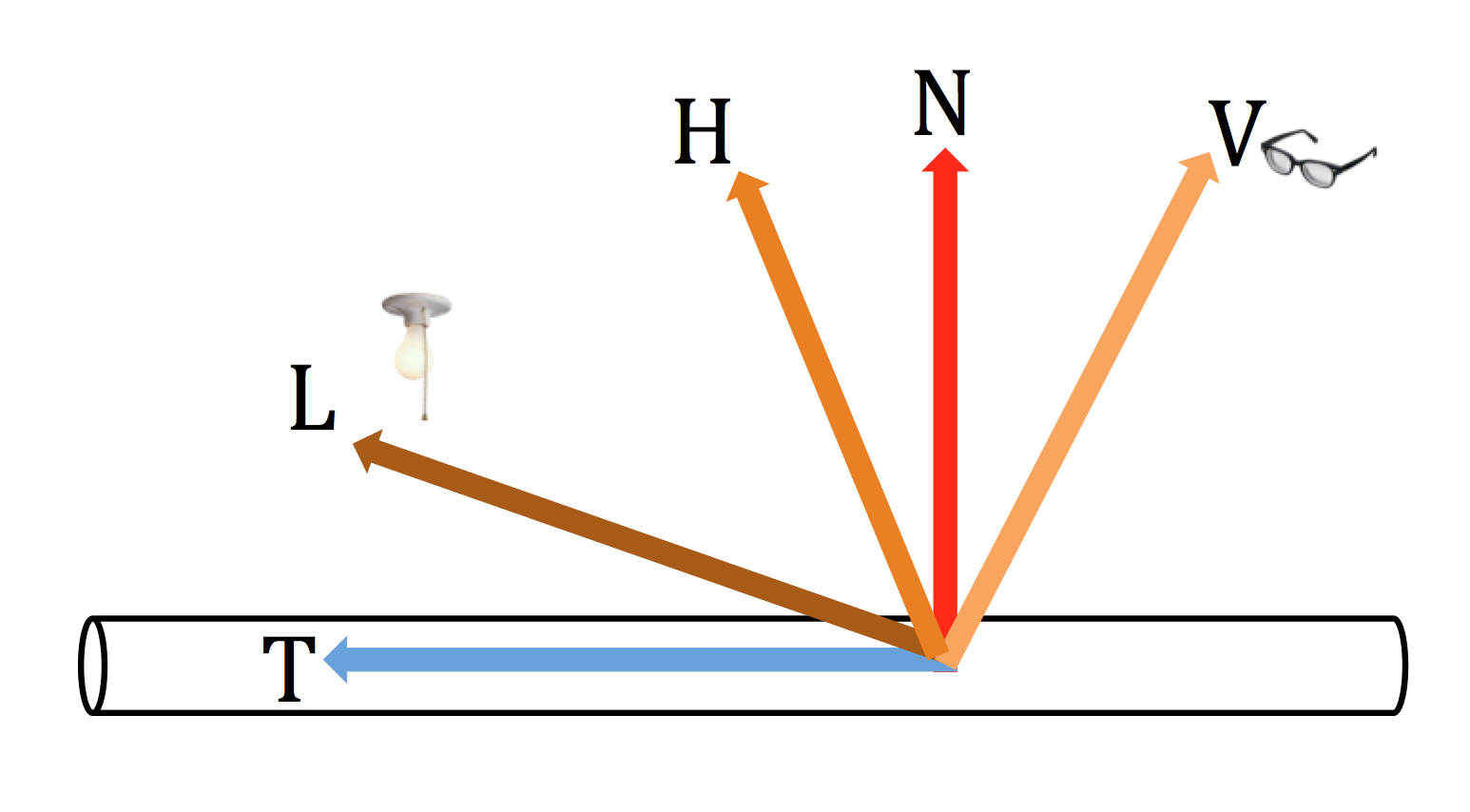T：切线向量。

L：光照向量。

H：L 和 V 的半角向量。H = normalize(L+V)。

N：法线向量。

V：视线向量。

\begin{aligned} 1 &= sin\theta^2 + cos\theta^2 \\ sin\theta^{specularity} &= \sqrt{1-cos\theta^2}^{specularity} \end{aligned}

$sin\theta$ $pow(sin\theta, specularity)$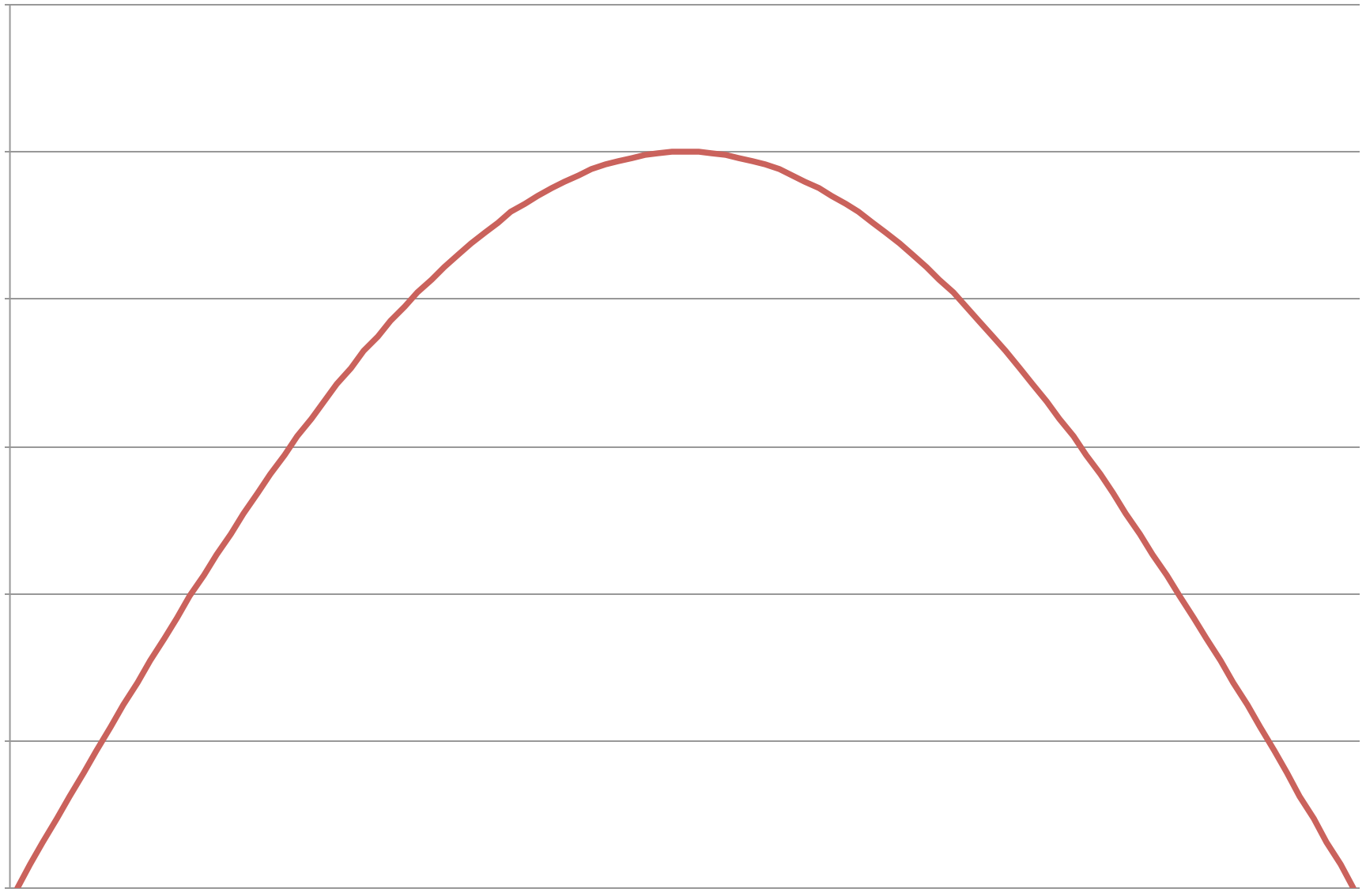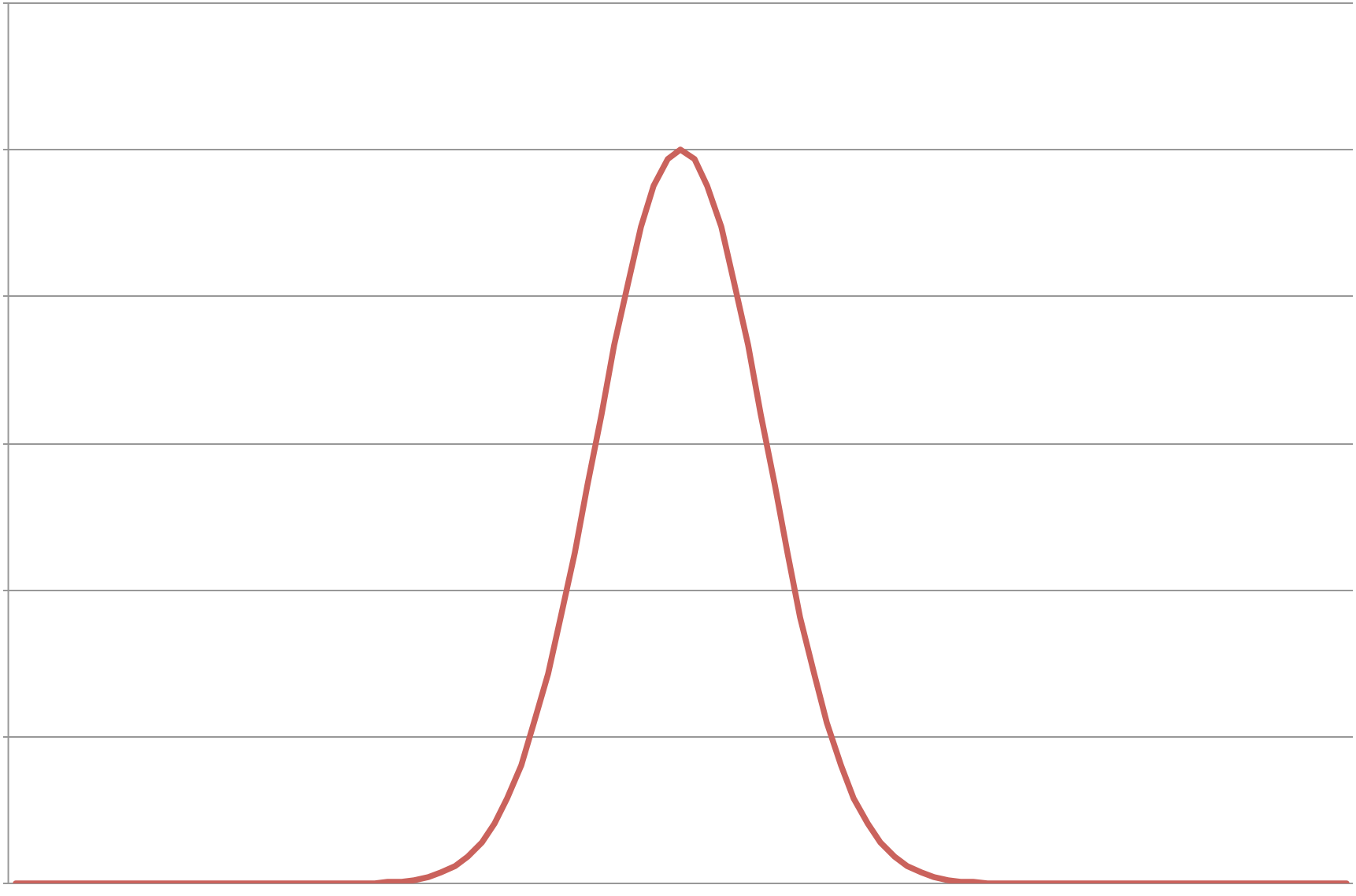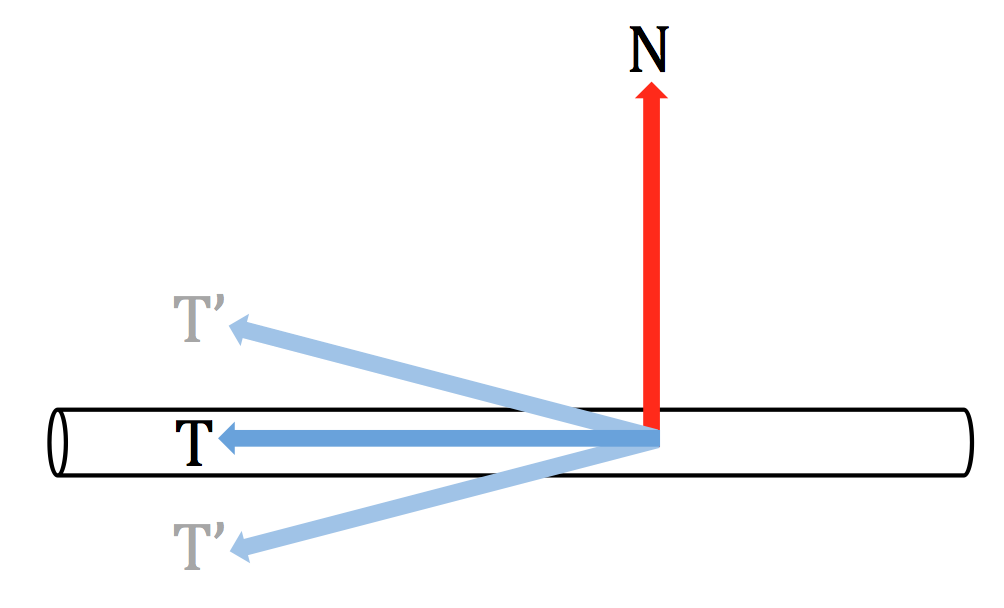float3 newT = normalize(T + shift * N);